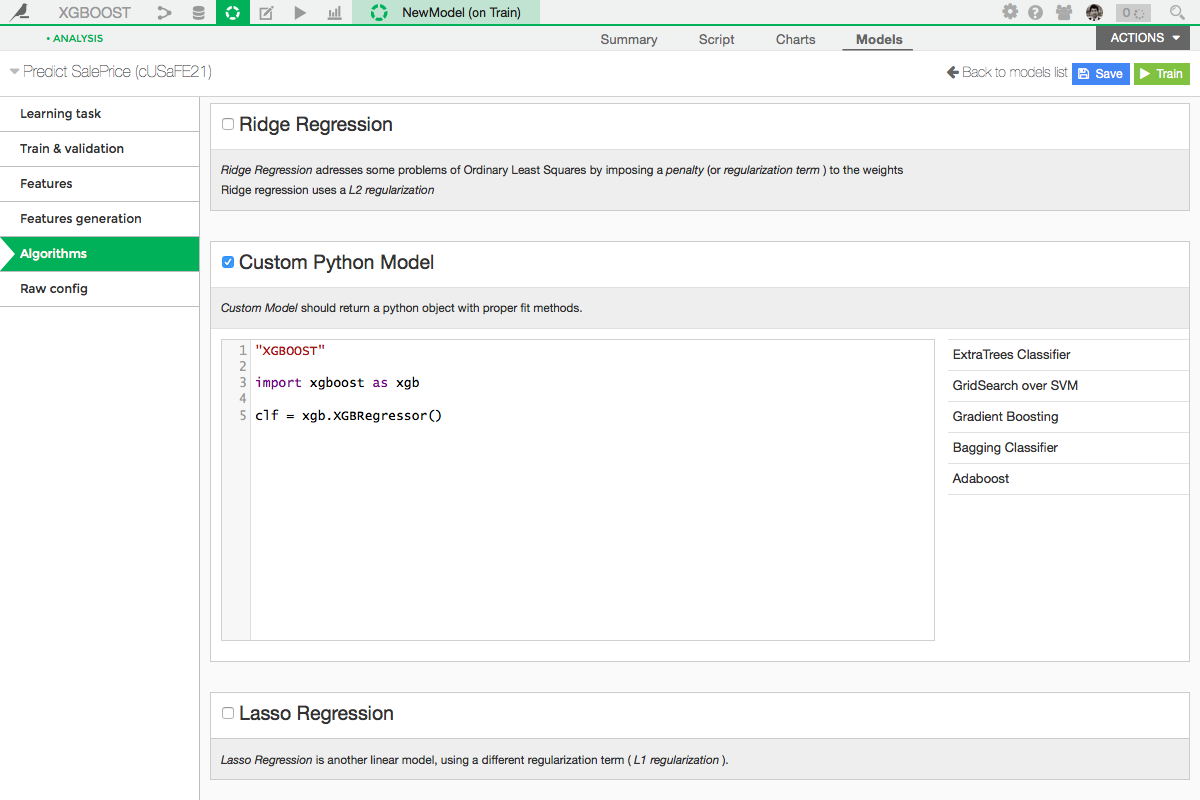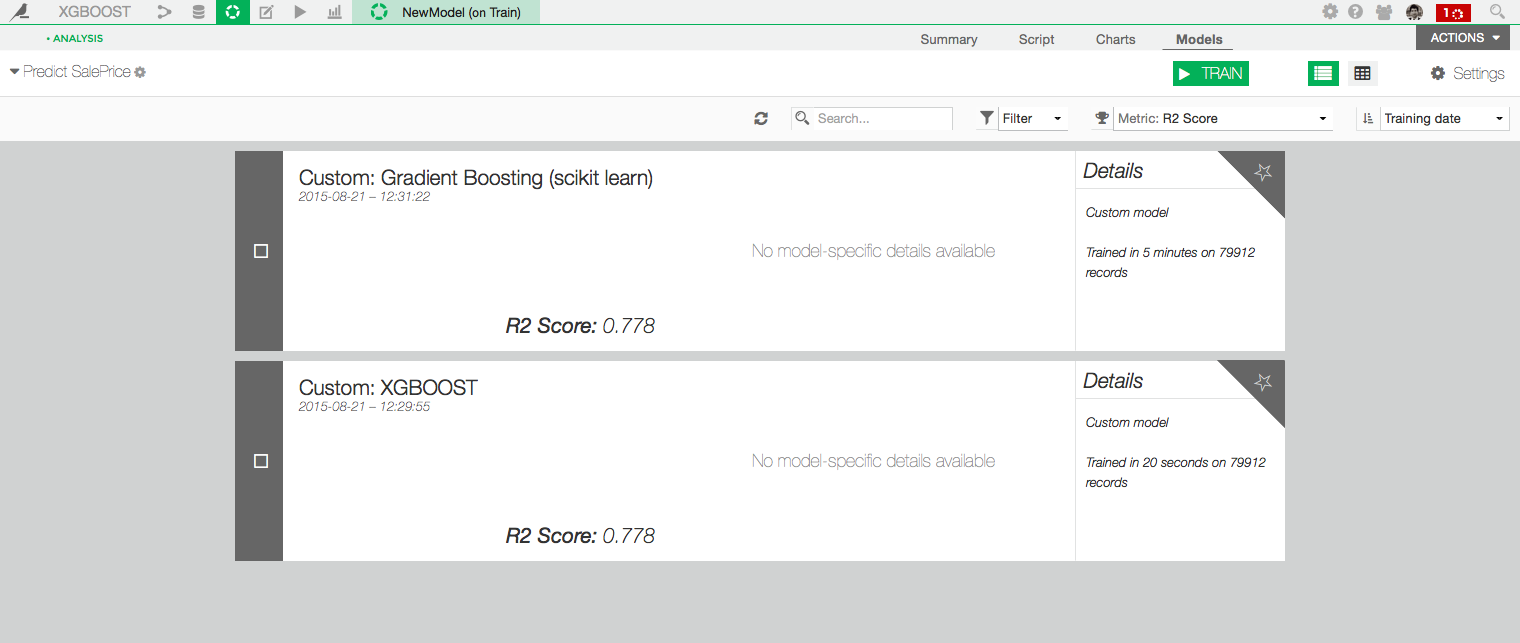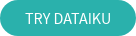XGBoost, you know this name if you're familiar with machine learning competitions. It's the algorithm you want to try: it's very fast, effective, easy to use, and comes with very cool features. If you're new to machine learning, check out this article on why algorithms are your friend. In this post, I'llI explain how you can use it in DSS without coding and some more advanced things you can do with Python code.

Since DSS 3.0, XGBoost has been natively integrated into DSS virtual machine learning, meaning you can train XGBoost models without writing any code.
You'll find more information about how to use XGBoost in visual machine learning in the reference documentation.

The rest of this post covers how to use XGBoost manually, for DSS 2.X

XGBoost is a gradient boosting tree method. Gradient, because it uses gradient descent, is a way to find a local minimum of a function: the algorithm follows the path of the descent. Boosting is a technique which is based on the fact that a set of weak learners is stronger than a single strong learner.  Explore Tianqi Chen presentation to grasp the theoretical aspect.

## Installation

XGBoost isn't in DSS by default, you have to get it on github, compile it, and install the python module on the python of DSS.

To check if everything works fine you can try to import xgboost in an ipython notebook.

## XGBoost in a custom model.

Take a dataset and in your analysis, go to Models / Settings / Algorithms and add a custom model. Here you'll have to write 3 lines of code to specify the model name, import xgboost and create your classifier:• XGBClassifier if your target binary.
• XGBRegressor if you do regression.

Let's compare it to scikit learn Gradient Boosting with both default parameter:Same R2 score but XGBoost was trained in 20 seconds against 5 minutes for the scikit learn GBT!

You can now deploy it like another model in DSS but maybe you'll want to change the default parameters to optimize your score!

## Parameters.

You can set some parameters to your XGBoost:

``````max_depth : int
Maximum tree depth for base learners.
learning_rate : float
Boosting learning rate (xgb's "eta")
n_estimators : int
Number of boosted trees to fit.
silent : boolean
Whether to print messages while running boosting.
objective : string
Specify the learning task and the corresponding learning objective.
Number of parallel threads used to run xgboost.
gamma : float
Minimum loss reduction required to make a further partition
on a leaf node of the tree.
min_child_weight : int
Minimum sum of instance weight(hessian) needed in a child.
max_delta_step : int
Maximum delta step we allow each tree's weight estimation to be.
subsample : float
Subsample ratio of the training instance.
colsample_bytree : float
Subsample ratio of columns when constructing each tree.
base_score:
The initial prediction score of all instances, global bias.
seed : int
Random number seed.
missing : float, optional
Value in the data which needs to be present as a missing value.
If None, defaults to np.nan.
``````

You have 2 ways to control overfitting in xgboost:

• Control the model complexity with max_depth, min_child_weight and gamma.
• Add randomness to make training robust to noise with subsample and colsample_bytree.

## Sparse matrix.

Xgboost can take in input sparse matrix. That's very useful because when you have categorical variables with high cardinality, you can convert them into dummies matrix without being out of memory!

For this, I use a python function:

This returns a sparse matrix of 3 columns, one by value of VAR_0001:

You can concatenate this matrix with other dummy matrices with the scipy hstack function:

## Early stopping.

A really cool feature is early stopping. As you are going to learn more and more trees, you will overfit your training dataset. Early stopping able you to specify a validation dataset and the number of iteration the algorithm should stop if the score on your validation dataset didn't increase.

To use it, you can specify in the fit method of the classifier an evaluation set, an evaluation method and the early stopping round number:

I set explicitly the n_estimators to a very large number. In your job log you'll see the score increasing on the dataset you put in the eval_set list:

## Features importance.

You can get the features importance easily in clf.booster().get_fscore()where clf is your trained classifier.

In a iPython notebook, I use this code to see it:## Hyperopt to gridsearch.

Fine-tuning your XGBoost can be done by exploring the space of parameters possibilities. For this task, I'm using the package Hyperopt. Hyperopt is a Python library for optimizing over awkward search spaces with real-valued, discrete, and conditional dimensions.

Here an exemple of a python recipe to use it:

This function trains a model, evaluates it and returns the error on the validation set. I define the space I want to explore, here I want to try values from 5 to 30 for max_depth, from 1 to 10 for min_child_weight and from 0.8 to 1 for subsample.

Hyperopt will minimize this error in a maximum of 100 experiments.

Here's more documentation: hyperopt

That's it! If you want to talk more about xgboost or anything related to data science, send me an email!